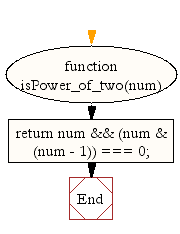# JavaScript: Return values that are powers of two

## JavaScript Math: Exercise-36 with Solution

Write a JavaScript function to return powers of two values.

Test Data :
console.log(isPower_of_two(64));
true
console.log(isPower_of_two(94));
false

Sample Solution:-

HTML Code:

``````<!DOCTYPE html>
<html>
<meta charset="utf-8">
<title>JavaScript function which will return values that are powers of two</title>
<body>

</body>
</html>
```
```

JavaScript Code:

``````function isPower_of_two(num)
{
return num && (num & (num - 1)) === 0;
}

console.log(isPower_of_two(64));
console.log(isPower_of_two(94));
```
```

Sample Output:

```true
false
```

Pictorial Presentation:Flowchart:Live Demo:

See the Pen javascript-math-exercise-36 by w3resource (@w3resource) on CodePen.

Improve this sample solution and post your code through Disqus

What is the difficulty level of this exercise?

Test your Programming skills with w3resource's quiz.

﻿